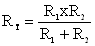#### Parallel Circuits Analysis

Parllel circuits have the following important characteristics.
Here is a typical parallel circuit with two loads (resistors) across the voltage supply.Circuit Legend:
•     VT is the Total Voltage
•     IT is the total Current
•     V1, and Vare the voltage drops across R1and R2
•     I1, and I2 are the currents through R1and R2
Parallel Circuits Facts:
Voltage: The voltage is always the same -- VT = V1 = V2
Current: The sum of all the branch currents (the current through each load) adds up to the total (source) current -- IT  = I1 + I2
Resistance: The Total resistance RT  can be calculated as follows:

• 1 / RT = 1 / R1 + 1 / R2
• There is also a convenient way to calculate  RT in the special case when you have only two resistors in parallel
•• RT  = VT / IT    (applying Ohm's Law)
Power: The Total Power PT  can be calculated in two possible ways:
1. PT = P1 + P2
2. PT  = VT  IT

Example:
In the circuit below, find all the unknown quantities:
 GIVEN FIND FIND VTotal  = VT = 40 V V2 = ? RT= ? ITotal  = IT= 8 A V1 = P1 = ? R2 = 5.0 Ω I1 = ? P2 = ? I2= 3 A R1 = ? PT = ?
Solution:
Use Ohm's law for each component.  Start with the component where you have two variables out of the three (R = VT / IT  )
(i)  Find  I1 If  I2= 3 A  and  I8A,  then  I1 =  I- I= 8 A - 3 A = 5 A
(ii) Find  R1      R1    VI1   = 40 V / 5 A = 8.0 Ω
(iii) Find V1    We know that V= V1 = V2    (In parallel circuits the voltage is always constant)
Therefore    V1= VT =  40V
(iv) Now we can find R 2
R 2 = V 2 / I  =  40V / 3A = 13.3Ω
(v) Find  the Total Resistance RT
1. Method #1 -----  1/RT = 1/R1 +1/ R2 = 1/ 8 Ω  + 1/13.3 Ω   = 0.20 Ω
Careful Now ... if  1/RT = 0.20 Ω   then, R= 1/ 0.20 Ω = 5.0 Ω

2. Method #2 ------ RT  = VT / IT      (Ohm's Law)
=  40 V / 8A  = 5.0 Ω
You can see that both methods yield the same result
(vi)   Find  P1       P1 =  V1 x  I= 40 V x 5 A = 200 W
This is the power used by R1
(vii)  Find  P2       P2 =  V2 x  I= 40 V x 3 A = 120 W
This is the power used by R2
(vii)   Find  PT       PT =  VT x  I= 40 V x 8 A = 320 W

This is the total power used by all three resistors together

Or, Alternatively, PT = P1 + P2  = 200 W + 120 W = 320 W
You can see that both methods yield the same results